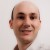# The Central Limit Theorem

Six Sigma – iSixSigma Forums Old Forums General The Central Limit Theorem

Viewing 2 posts - 1 through 2 (of 2 total)
• Author
Posts
• #33684

What should we do if the data is non-normal distribution when we do SPC analysis? Someone tell me it is no problem because of  The Central Limit Theorem. Thanks!

0
#91505Gabriel
Participant

As allways, the answer is “it depends”.
The central limit theorem says that the average of samples of size n taken from a population of individuals will tend to be normally distributed as n increases. Depending on how far from the normal is the shape of distribution of individuals, it will take a larger or a smaller n to reach a point where the ditribution of averages matches pretty well the normal distribution. n=30 works well even for most not-normal distributions. n=3 to 5 works well for most nearly symmetical distributions.
Now we have to link all this with SPC. If you are charting Xbar, then you are charting “averages of samples of size n” (subgroup averages). Xbar values will be more or less normally distributed depending on how far from normal is the original distribution and on the subgroup size (n).
If you are charting individual values, or if you have a combination of a too skewed distribution and a small subgroup size, then the central limit theorem won’t help to normalize the distribution.
However, note that a lot of non-normal parameters are charted all the time. Ranges, standard deviations, fraction non conforming are all intrinsically not normal. However they are charted and the control limits are set at ±3 sigmas as if they were normal. I never heard someone questioning if he/she can chart ranges teven when they are not normal.
Returning to the Xbar case, even if the distribution of Xbar gets normal thanks to the central limit theorem, there is still an issue to think about. How the control limits are set. The control limits are to be set at ±3sigmas of the charted variable (in this case ±3*sigma(Xbar)). Again thanks to the central limit theorem, sigma(Xbar)=sigma(X)/sqrt(n). The problem is in the estimation of sigma(X). If you are using an R chart together with the Xbar chart, the typical estimation of sigma(X) is Rbar/d2. Note that d2 is the proportionality constant that links Rbar with sigma(X) FOR NORMAL DISTRIBUTIONS which, as it was assumed, it is not the case. The factor A2 used to calculate the control lims are based on Rbar/d2: A2=3*[Rbar/d2]/sqrt(n). A2 will be biased from the desired ±3sigma(Xbar) if the distribution of X is not normal. I was told that Rbar/d2, even when it was defined for normal distributions, is pretty robust for other not-normal distributions too as an estimation of sigma(X). If you are using an Xbar-S chart instead of an Xbar-R chart, the same happens with the constant c4.
I used Xbar-R charts with distributions that were known to be not normal, and used them without any special consideration, and encountered no problems related to the lack of normality. My advice would be: Keep it simple. Use the control chart without careing about the shape of the distribution unless you have some solid reasons not to do so or you encounter problems during the implementation due the lack of normality.
Another issue to touch is tha capabilty indexes, such as Cp/Cpk, which can be part of what you describe as “SPC analysis”.
Here again, the answer is “it dependes”.
If you use Cp/Cpk to estimate an absolute reference of the actual process capability (for example you relate it to actual deffectives rates, or you want to see if you meet a customer requierment) then normality matters. You should either normalize the data or use the definitions of Cp/Cpk based on percentiles and not on the average and standard deviation.
But if you use it to monitor the evolution of the process capability and check the effectiveness of improvement efforts, then the shape does not matter. You just use the first Cpk as a baseline and then compare the fututre results. Regardless of the shape of the ditribution, if the capability index improved, then your process improved.
Hope it helped.

0
Viewing 2 posts - 1 through 2 (of 2 total)

The forum ‘General’ is closed to new topics and replies.